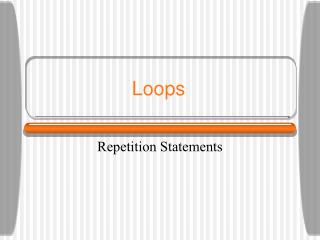DownloadDownload PresentationLoops

# Loops

Download Presentation## Loops

- - - - - - - - - - - - - - - - - - - - - - - - - - - E N D - - - - - - - - - - - - - - - - - - - - - - - - - - -
##### Presentation Transcript

1. Loops Repetition Statements

2. 0 Repetition Statements • Repetition statements allow us to execute a statement multiple times • Often they are referred to as loops • Like conditional statements, they are controlled by boolean expressions

3. 0 Repetition Statements • Java has three kinds of repetition statements: • the while loop • the do loop • the for loop • The programmer should choose the right kind of loop for the situation

4. 0 The while Statement syntax • A while statement has the following syntax: while ( condition ){ statements; } If the condition is true, the statement is executed Then the condition is evaluated again, and if it is still true, the statement is executed again The statement is executed repeatedly until the condition becomes false

5. condition evaluated true false statement 0 Logic of a while Loop

6. 0 Example 1: • An example of a while statement: int count = 1; while (count <= 3){ System.out.println (count); count++; }

7. 0 Example 2 • An example of a while statement: int count = 1; while (count < 2){ System.out.println (count); count++; }

8. 0 Example 3 • An example of a while statement: int count = 7; while (count < 7){ System.out.println (count); count++; }

9. 0 Example 4 • An example of a while statement: int count = 2; while (count < 3){ System.out.println (count); } Infinite Loop

10. Infinite Loops • The body of a while loop eventually must make the condition false • If not, it is called an infinite loop, which will execute until the user interrupts the program • This is a common logical error • You should always double check the logic of a program to ensure that your loops will terminate normally Java/loops/While1.java WhileEx2.java WhileEx1.java

11. Nested Loops • Similar to nested if statements, loops can be nested as well • That is, the body of a loop can contain another loop • For each iteration of the outer loop, the inner loop iterates completely

12. Nested Loops • How many times will the string "Here" be printed? count1 = 1; while (count1 <= 10){ count2 = 1; while (count2 <= 20){ System.out.println ("Here"); count2++; } count1++; } 10 * 20 = 200

13. The do Statement • A do statement has the following syntax: do{ statement; } while ( condition ) The statement is executed once initially, and then the condition is evaluated The statement is executed repeatedly until the condition becomes false

14. statement true condition evaluated false Logic of a do Loop

15. The do Statement • An example of a do loop: int count = 0; do{ count++; System.out.println (count); } while (count < 3);

16. The do Statement • An example of a do loop: int count = 100; do{ count++; System.out.println (count); } while (count < 3); The body of a do loop executes at least once

17. The while Loop The do Loop condition evaluated statement true true false condition evaluated statement false Comparing while and do

18. The statement is executed until the condition becomes false The initialization is executed once before the loop begins The increment portion is executed at the end of each iteration The for Statement • A for statement has the following syntax: for ( initialization ; condition ; update ){ statements; }

19. initialization condition evaluated true false statements update Logic of a for loop

20. The for Statement • A for loop is functionally equivalent to the following while loop structure: initialization; while ( condition ){ statement; update; }

21. The for Statement • An example of a for loop: for (int count=1; count <= 3; count++){ System.out.println (count); } The initialization section can be used to declare a variable Like a while loop, the condition of a for loop is tested prior to executing the loop body Therefore, the body of a for loop will execute zero or more times

22. The for Statement • Each expression in the header of a for loop is optional • If the initialization is left out, no initialization is performed • If the condition is left out, it is always considered to be true, and therefore creates an infinite loop • If the increment is left out, no increment operation is performed Java/Loops.For1.java For2.java, For3.java, For4.java,

23. Questions??? for do while Java/Loops/ForWhile.java ForInsideWhile.java